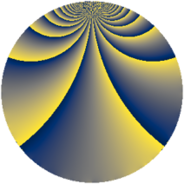# Properties

 Label 1045.2.nLevel $1045$ Weight $2$ Character orbit 1045.n Rep. character $\chi_{1045}(191,\cdot)$ Character field $\Q(\zeta_{5})$ Dimension $288$ Sturm bound $240$

# Related objects

## Defining parameters

 Level: $$N$$ $$=$$ $$1045 = 5 \cdot 11 \cdot 19$$ Weight: $$k$$ $$=$$ $$2$$ Character orbit: $$[\chi]$$ $$=$$ 1045.n (of order $$5$$ and degree $$4$$) Character conductor: $$\operatorname{cond}(\chi)$$ $$=$$ $$11$$ Character field: $$\Q(\zeta_{5})$$ Sturm bound: $$240$$

## Dimensions

The following table gives the dimensions of various subspaces of $$M_{2}(1045, [\chi])$$.

Total New Old
Modular forms 496 288 208
Cusp forms 464 288 176
Eisenstein series 32 0 32

## Trace form

 $$288 q + 8 q^{2} - 64 q^{4} + 8 q^{7} - 16 q^{8} - 84 q^{9} + O(q^{10})$$ $$288 q + 8 q^{2} - 64 q^{4} + 8 q^{7} - 16 q^{8} - 84 q^{9} - 4 q^{11} - 12 q^{13} - 4 q^{14} - 12 q^{15} - 48 q^{16} + 24 q^{17} - 28 q^{18} + 8 q^{20} + 64 q^{21} - 72 q^{22} + 48 q^{23} - 56 q^{24} - 72 q^{25} - 24 q^{26} - 36 q^{27} + 4 q^{28} + 40 q^{29} + 24 q^{30} + 16 q^{32} + 36 q^{33} + 32 q^{34} + 16 q^{35} - 24 q^{36} - 72 q^{37} - 24 q^{39} - 28 q^{41} - 32 q^{43} + 44 q^{44} + 4 q^{46} - 40 q^{47} - 48 q^{49} + 8 q^{50} - 24 q^{51} + 80 q^{52} - 80 q^{53} - 8 q^{54} - 24 q^{55} - 16 q^{56} + 8 q^{57} + 44 q^{58} + 56 q^{59} - 52 q^{60} - 32 q^{61} + 32 q^{62} - 92 q^{63} + 32 q^{64} + 8 q^{65} - 148 q^{66} + 56 q^{67} - 76 q^{68} + 112 q^{69} + 8 q^{70} - 48 q^{71} - 20 q^{72} - 28 q^{73} - 116 q^{74} + 104 q^{77} + 88 q^{78} - 72 q^{79} - 8 q^{80} - 76 q^{81} + 64 q^{82} + 20 q^{83} - 184 q^{84} + 32 q^{85} + 72 q^{86} + 24 q^{87} - 4 q^{88} + 56 q^{89} - 124 q^{91} - 248 q^{92} + 48 q^{93} - 64 q^{94} + 16 q^{95} + 84 q^{96} - 60 q^{97} + 192 q^{98} - 24 q^{99} + O(q^{100})$$

## Decomposition of $$S_{2}^{\mathrm{new}}(1045, [\chi])$$ into newform subspaces

The newforms in this space have not yet been added to the LMFDB.

## Decomposition of $$S_{2}^{\mathrm{old}}(1045, [\chi])$$ into lower level spaces

$$S_{2}^{\mathrm{old}}(1045, [\chi]) \cong$$ $$S_{2}^{\mathrm{new}}(55, [\chi])$$$$^{\oplus 2}$$$$\oplus$$$$S_{2}^{\mathrm{new}}(209, [\chi])$$$$^{\oplus 2}$$